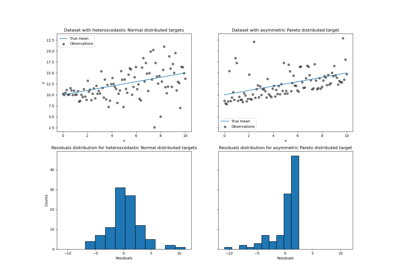# sklearn.linear_model.QuantileRegressor¶

class sklearn.linear_model.QuantileRegressor(*, quantile=0.5, alpha=1.0, fit_intercept=True, solver='warn', solver_options=None)[source]

Linear regression model that predicts conditional quantiles.

The linear QuantileRegressor optimizes the pinball loss for a desired quantile and is robust to outliers.

This model uses an L1 regularization like Lasso.

Read more in the User Guide.

New in version 1.0.

Parameters:
quantilefloat, default=0.5

The quantile that the model tries to predict. It must be strictly between 0 and 1. If 0.5 (default), the model predicts the 50% quantile, i.e. the median.

alphafloat, default=1.0

Regularization constant that multiplies the L1 penalty term.

fit_interceptbool, default=True

Whether or not to fit the intercept.

solver{‘highs-ds’, ‘highs-ipm’, ‘highs’, ‘interior-point’, ‘revised simplex’}, default=’interior-point’

Method used by scipy.optimize.linprog to solve the linear programming formulation.

From scipy>=1.6.0, it is recommended to use the highs methods because they are the fastest ones. Solvers “highs-ds”, “highs-ipm” and “highs” support sparse input data and, in fact, always convert to sparse csc.

From scipy>=1.11.0, “interior-point” is not available anymore.

Changed in version 1.4: The default of solver will change to "highs" in version 1.4.

solver_optionsdict, default=None

Additional parameters passed to scipy.optimize.linprog as options. If None and if solver='interior-point', then {"lstsq": True} is passed to scipy.optimize.linprog for the sake of stability.

Attributes:
coef_array of shape (n_features,)

Estimated coefficients for the features.

intercept_float

The intercept of the model, aka bias term.

n_features_in_int

Number of features seen during fit.

New in version 0.24.

feature_names_in_ndarray of shape (n_features_in_,)

Names of features seen during fit. Defined only when X has feature names that are all strings.

New in version 1.0.

n_iter_int

The actual number of iterations performed by the solver.

Lasso

The Lasso is a linear model that estimates sparse coefficients with l1 regularization.

HuberRegressor

Linear regression model that is robust to outliers.

Examples

>>> from sklearn.linear_model import QuantileRegressor
>>> import numpy as np
>>> n_samples, n_features = 10, 2
>>> rng = np.random.RandomState(0)
>>> y = rng.randn(n_samples)
>>> X = rng.randn(n_samples, n_features)
>>> # the two following lines are optional in practice
>>> from sklearn.utils.fixes import sp_version, parse_version
>>> solver = "highs" if sp_version >= parse_version("1.6.0") else "interior-point"
>>> reg = QuantileRegressor(quantile=0.8, solver=solver).fit(X, y)
>>> np.mean(y <= reg.predict(X))
0.8


Methods

 fit(X, y[, sample_weight]) Fit the model according to the given training data. get_params([deep]) Get parameters for this estimator. Predict using the linear model. score(X, y[, sample_weight]) Return the coefficient of determination of the prediction. set_params(**params) Set the parameters of this estimator.
fit(X, y, sample_weight=None)[source]

Fit the model according to the given training data.

Parameters:
X{array-like, sparse matrix} of shape (n_samples, n_features)

Training data.

yarray-like of shape (n_samples,)

Target values.

sample_weightarray-like of shape (n_samples,), default=None

Sample weights.

Returns:
selfobject

Returns self.

get_params(deep=True)[source]

Get parameters for this estimator.

Parameters:
deepbool, default=True

If True, will return the parameters for this estimator and contained subobjects that are estimators.

Returns:
paramsdict

Parameter names mapped to their values.

predict(X)[source]

Predict using the linear model.

Parameters:
Xarray-like or sparse matrix, shape (n_samples, n_features)

Samples.

Returns:
Carray, shape (n_samples,)

Returns predicted values.

score(X, y, sample_weight=None)[source]

Return the coefficient of determination of the prediction.

The coefficient of determination $$R^2$$ is defined as $$(1 - \frac{u}{v})$$, where $$u$$ is the residual sum of squares ((y_true - y_pred)** 2).sum() and $$v$$ is the total sum of squares ((y_true - y_true.mean()) ** 2).sum(). The best possible score is 1.0 and it can be negative (because the model can be arbitrarily worse). A constant model that always predicts the expected value of y, disregarding the input features, would get a $$R^2$$ score of 0.0.

Parameters:
Xarray-like of shape (n_samples, n_features)

Test samples. For some estimators this may be a precomputed kernel matrix or a list of generic objects instead with shape (n_samples, n_samples_fitted), where n_samples_fitted is the number of samples used in the fitting for the estimator.

yarray-like of shape (n_samples,) or (n_samples, n_outputs)

True values for X.

sample_weightarray-like of shape (n_samples,), default=None

Sample weights.

Returns:
scorefloat

$$R^2$$ of self.predict(X) wrt. y.

Notes

The $$R^2$$ score used when calling score on a regressor uses multioutput='uniform_average' from version 0.23 to keep consistent with default value of r2_score. This influences the score method of all the multioutput regressors (except for MultiOutputRegressor).

set_params(**params)[source]

Set the parameters of this estimator.

The method works on simple estimators as well as on nested objects (such as Pipeline). The latter have parameters of the form <component>__<parameter> so that it’s possible to update each component of a nested object.

Parameters:
**paramsdict

Estimator parameters.

Returns:
selfestimator instance

Estimator instance.

## Examples using sklearn.linear_model.QuantileRegressor¶Quantile regression

Quantile regression